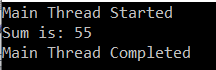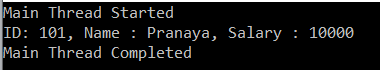## C# Task Return Value with Examples

In this article, I am going to discuss the C# Task return value in detail. Please read our previous article where we discussed how to create and use the task object in C# in different ways. At the end of this article, you will understand How to Return a Value from a Task in C# with examples.

The .NET Framework also provides a generic version of the Task class i.e. Task<T>. Using this Task<T> class we can return data or value from a task. In Task<T>, T represents the data type that you want to returns as a result of the task.

##### Example:

In the following example, the CalculateSum method takes an input integer value and calculates the sum of the number starting from 1 to that number. Here the CalculateSum method returns a double value. As the return value from the CalculateSum method is of double type, so here we need to use Task<double> as shown in the below example.

```using System;

{
class Program
{
static void Main(string[] args)
{

{
return CalculateSum(10);
});

}

static double CalculateSum(int num)
{
double sum = 0;
for (int count = 1; count <= num; count++)
{
sum += count;
}
return sum;
}
}
}
```

Output:Note: The Result property of the Task object blocks the calling thread until the task finishes its work.

##### Example:

In the below example, we are writing the logic as part of the Anonymous method.

```using System;
{
class Program
{
static void Main(string[] args)
{

{
double sum = 0;
for (int count = 1; count <= 10; count++)
{
sum += count;
}
return sum;
});

}
}
}```

It will also give you the same output as the previous example. So, whenever your logic is a few lines and that is going to be used only once, then it is always better to write the logic with the anonymous method.

##### Example: Returning Complex Type Value From a task

In the below example, we are returning a Complex type.

```using System;

{
class Program
{
static void Main(string[] args)
{
{
Employee employee = new Employee()
{
ID = 101,
Name = "Pranaya",
Salary = 10000
};

return employee;
});

Console.WriteLine(\$"ID: {emp.ID}, Name : {emp.Name}, Salary : {emp.Salary}");
}
}

public class Employee
{
public int ID { get; set; }
public string Name { get; set; }
public double Salary { get; set; }
}
}
```
###### Output:That’s it for today. In the next article, I am going to discuss Chaining Tasks by Using Continuation Tasks in C# with some examples. Here, in this article, I try to explain the C# Task return value i.e. how to return a value from a task in C# with examples. I hope you enjoy this article.